TitleGRE Math Practice Test II
P. 1 (Problems 1 - 9)

WTAMU > Virtual Math Lab > GRE MathIntroduction

 If this is your first visit to our website, please read the disclaimer. Because of the enormity of this part, I split the adaptive test into three pages.  This page, P. 1, has the questions to all levels of the first 9 problems. P. 2 has the questions to all levels of problems 10 - 19. P. 3 has the questions to all levels of problems 20 - 28. As you click on the links for this test, it will take you to the next page at the appropriate time. Printing warning: Note that because of all of the levels per problem, there are a lot of questions on this page.  So if you wish to print this out, note that there will be a lot of pages to print.

THE REAL GRE TEST THAT YOU WILL BE TAKING ON THE COMPUTER IS AN ADAPTIVE TEST:

 Adaptive means that the level of the questions that you get are dependent on if you answer previous questions correctly or not.  The higher the level of question the higher the overall weight that problem has.  Also note that the design of the test is different from this practice test.  All the problems on this test are on the same page, though as you click on your answer, it will bring you to the appropriate next problem.  On the GRE test you will be taking, one problem at a time will appear on your computer screen.  When a problem appears, you will click on the answer at which point it proceeds to the next problem.

IF THIS IS YOUR FIRST VISIT TO THIS WEBPAGE, IT IS IMPORTANT THAT YOU READ THIS SECTION!!

HOW TO EFFECTIVELY USE THIS WEBPAGE:Practice Test II, Problems 1 -9

 Everyone needs to start with 1 - 3 (problem 1, level 3).  Again, make sure you mark the problem, level, and answer to each question on a sheet of paper.  Click on the letter of your answer and it will bring you to the next appropriate question.

 1 - 3.

 Column A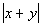Column BA)  Column A’s quantity is greater. B)  Column B’s quantity is greater. C)  The quantities are the same. D)  The relationship cannot be determined from the information given.

 2 - 2.

 Column A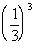Column B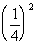A)  Column A’s quantity is greater. B)  Column B’s quantity is greater. C)  The quantities are the same. D)  The relationship cannot be determined from the information given.

 2 - 4.

 Column A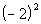Column B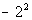A)  Column A’s quantity is greater. B)  Column B’s quantity is greater. C)  The quantities are the same. D)  The relationship cannot be determined from the information given.

 3 - 1.  A cylindrical building contains wheat.  The building has a radius of 20 feet and a depth of 50 feet.  What is the volume of this cylindrical building?

 A)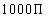square feet  B)cubic feet  C)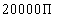square feet  D)cubic feet  E)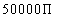cubic feet

 3 - 3.  A cylindrical building contains wheat.  The building has a diameter of 17 feet and a depth of 40 feet.  What is the volume of this cylindrical building?

 A)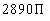square feet  B)cubic feet  C)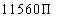square feet  D)cubic feet  E)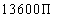cubic feet

 3 - 5.  Two identical cylindrical buildings contain wheat.  Each building has a diameter of 21 feet and a depth of 30 feet.  If one cubic foot of wheat weighs 7 pounds, approximately how many pounds of wheat can be stored if both building are full.

 A)   46,305  B)  290,795  C)   581,591  D)  72,699  E)   145,398

 4 - 1.

 Column A 30% of 50,000 Column B 1500

 A)  Column A’s quantity is greater.  B)  Column B’s quantity is greater.  C)  The quantities are the same.  D)  The relationship cannot be determined from the information given.

 4 - 2.

 Column A 7% of 25,000 Column BA)  Column A’s quantity is greater.  B)  Column B’s quantity is greater.  C)  The quantities are the same.  D)  The relationship cannot be determined from the information given.

 4 - 4.

 Column A 7.5% of 8000 Column B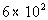A)  Column A’s quantity is greater.  B)  Column B’s quantity is greater.  C)  The quantities are the same.  D)  The relationship cannot be determined from the information given.

 4- 5.

 Column A .065% of .4 Column B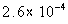A)  Column A’s quantity is greater.  B)  Column B’s quantity is greater.  C)  The quantities are the same.  D)  The relationship cannot be determined from the information given.

 5 - 1.  If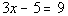, what is the value of x?

 A)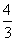B)  4  C)D)  11  E)   17

 5 - 2.  If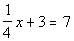, what  is the value of x?

 A)   0  B)  1  C)D)  16  E)   40

 5 - 3.  If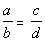and a, b, c, and d are positive, then which of the following has to be true?

 A)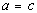B)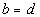C)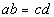D)E)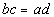5 - 4.  If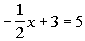, what is the value of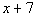?

 A)   -4  B)    3  C)    4  D)   11  E)   16

 5 - 5.  If, what is the value of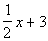?

 A)   -4.5  B)   -15  C)     4.5  D)    15  E)    -27

 6 - 1.

 Column A Two different coins are tossed.  The number of possible outcomes  of tossing the two coins. Column B 4

 A)  Column A’s quantity is greater.  B)  Column B’s quantity is greater.  C)  The quantities are the same.  D)  The relationship cannot be determined from the information given.

 6 - 2.

 Column A A combo special includes one sandwich,  one bag of chips and one drink.  There are four types of sandwiches,  three types of chips and three types of drinks.  The number of combos possible. Column B 24

 A)  Column A’s quantity is greater.  B)  Column B’s quantity is greater.  C)  The quantities are the same.  D)  The relationship cannot be determined from the information given.

 6 - 3.

 Column A The number of ways to arrange  five CD’s on a shelf. Column B 125

 A)  Column A’s quantity is greater.  B)  Column B’s quantity is greater.  C)  The quantities are the same.  D)  The relationship cannot be determined from the information given.

 6 - 4.

 Column A Probability of randomly selecting a given  name out of a hat containing  fifteen different names. Column B .07

 A)  Column A’s quantity is greater.  B)  Column B’s quantity is greater.  C)  The quantities are the same.  D)  The relationship cannot be determined from the information given.

 6 - 5.

 Column A A bag contains four red, twelve green,  and 14 black jelly beans.  The probability that a red one is not picked. Column B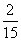A)  Column A’s quantity is greater.  B)  Column B’s quantity is greater.  C)  The quantities are the same.  D)  The relationship cannot be determined from the information given.

 7 - 1.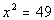Column A x Column B 7

 A)  Column A’s quantity is greater.  B)  Column B’s quantity is greater.  C)  The quantities are the same.  D)  The relationship cannot be determined from the information given.

 7 - 2.Column A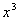Column B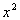A)  Column A’s quantity is greater.  B)  Column B’s quantity is greater.  C)  The quantities are the same.  D)  The relationship cannot be determined from the information given.

 7 - 3.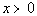Column AColumn BA)  Column A’s quantity is greater.  B)  Column B’s quantity is greater.  C)  The quantities are the same.  D)  The relationship cannot be determined from the information given.

 7 - 4.  x is a positive integer: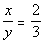Column A y Column B 1

 A)  Column A’s quantity is greater.  B)  Column B’s quantity is greater.  C)  The quantities are the same.  D)  The relationship cannot be determined from the information given.

 7 - 5.Column AColumn B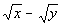A)  Column A’s quantity is greater.  B)  Column B’s quantity is greater.  C)  The quantities are the same.  D)  The relationship cannot be determined from the information given.

 8 - 1.In the figure above, if XY is a line segment, what is the number of degrees in the sum of a + b?  a and b are measured in degrees.

 A)  40  B)  50  C)  90  D)  130  E)  180

 8 - 2.In the figure above, if AB is a straight line and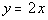, then how many degrees is y?  x and y are measured in degrees.

 A)   60  B)  90  C)  120  D)  180  E)   Not enough information to answer this question.

 8 - 3.In the figure above, if XY is a line segment, how many degrees is 4a?  a and b are measured in degrees.

 A)  4  B)  60  C)  144  D)  240  E)  Not enough information to answer this question.

 8 - 4.In the figure above, if XY is a line segment, what is the value of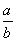?  a and b are measured in degrees.

 A)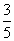B)C)   1  D)E)   Not enough information to answer this question.

 8 - 5.In the figure above, if XY is a line segment, what is the value of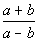?  a and b are measured in degrees.

 A)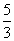B)  -4  C)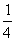D)    4  E)    Not enough information to answer this question.

 9 - 1.

 Column A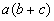Column B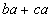A)  Column A’s quantity is greater.  B)  Column B’s quantity is greater.  C)  The quantities are the same.  D)  The relationship cannot be determined from the information given.

 9 - 2.

 Column A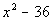Column BA)  Column A’s quantity is greater.  B)  Column B’s quantity is greater.  C)  The quantities are the same.  D)  The relationship cannot be determined from the information given.

 9 - 3.

 Column A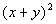Column BA)  Column A’s quantity is greater.  B)  Column B’s quantity is greater.  C)  The quantities are the same.  D)  The relationship cannot be determined from the information given.

 9 - 4.

 Column A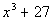Column B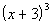A)  Column A’s quantity is greater.  B) Column B’s quantity is greater.  C) The quantities are the same.  D) The relationship cannot be determined from the information given.

 9 - 5.

 Column A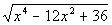Column B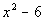A) Column A’s quantity is greater.  B) Column B’s quantity is greater.  C) The quantities are the same. D) The relationship cannot be determined from the information given.

 Disclaimer: Note that we can not guarantee that you will pass your test after going through any of the tutorials in this website.   However, it will definitely help you to better understand the topics covered.  WTAMU and Kim Seward are not responsible for how a student does on any test for any reason including not being able to access the website due to any technology problems.  GRE and Graduate Record Examination are the registered trademarks of Educational Testing Service (ETS). The material here has neither been reviewed nor endorsed by ETS.  Throughout this website, we link to various outside sources.  WTAMU and Kim Seward do not have any ownership to any of these outside websites and cannot give you permission to make any kind of copies of anything  found at any of these websites that we link to.  It is purely for you to link to for information or fun as you go through the study session.  Each of these websites have a copy right clause that you need to read carefully if you are wanting to do anything other than go to the website and read it.  We discourage any illegal use of the webpages found at these sites.
 All contents copyright (C) 2003 - 2008, WTAMU and Kim Seward. All rights reserved.  Last revised on September 29, 2008 by Kim Seward.